# Run Python in the Browser! | PyScript tutorial - mr parui web

A First Look at PyScript: Python in the Web Browser PyScript is a framework that allows users to create rich Python applications in the browser

So here I'm, a little bit late to the latest update. Still, I figured today I'd share my thoughts on py script and run you through a few quick demos of how you use this in your HTML code. Now, if you're unfamiliar with py script, this is something that is brand new so let's get started with this new blog.

From what I know, it came out a couple of months ago. I just heard about it the last month ago, so I'm writing this blog to make you aware of what is coming new in the market. This blog lets you inject python code and run it in the browser in your HTML document.

## Features of the py script

here are the some features of the py script offers

• Now you can import python files
• you can import Python modules.
• You can display a matplotlib graph directly on the HTML page
• generate random numbers.
• You can get values from input fields or change document tags. etc
• lastly you can use the python on the web itself

It's pretty cool even in the very early and alpha stage that it's in right now, and I think it's something we can hopefully look forward to in the future; with that said, though, let me hop over to the visual studio code I'll give you a few quick demos hopefully you'll get a sense of what this can do.

## Setting up the py script dev env.

This is super simple to set up, so this is a super exciting project, and today we have a first look at it py script gets developed by the people from anaconda and now before I show you how to use it

let's quickly go over the features that py script offers first of all it allows us to run python in the browser so it enables drop in content external file hosting and application hosting without the reliance on server side configuration so this is just html in the end then we can use the whole python ecosystem so of course we can use all core modules but we can also run many popular packages of python and the scientific stack such as numpy pandas scikit-learn and more this is super cool for machine learning and data science websites.

here are the main html tags that will be using to creating the code

<py-script> , <py-env> , <py-repl>

## what will I cover about pyscript tutorial ?

Now I'll show you an example we can very quickly show matplotlib in the browser then we can combine it with javascript so we have bi-directional communication between python and javascript objects and with this we can for example very efficiently run a Mario game in the browser which I show you at the end then we get environment management that is repl on the py script

So it allows users to define what packages and files to include we get a visual application development so we can use readily available curated UI components such as buttons containers text boxes and more..

we have a very flexible framework so a flexible framework that can be leveraged to create and share newly pluggable and extensible components directly in python.

So this all sounds super exciting and now let's have a look at how to use it so let's have a look at how to get started so we could download it and compile it ourselves for this we need node.js or we can simply click on install and then

we get just kidding you don't need to install anything so we just have to include one CSS file and one javascript file and this is hosted for us so we can copy this code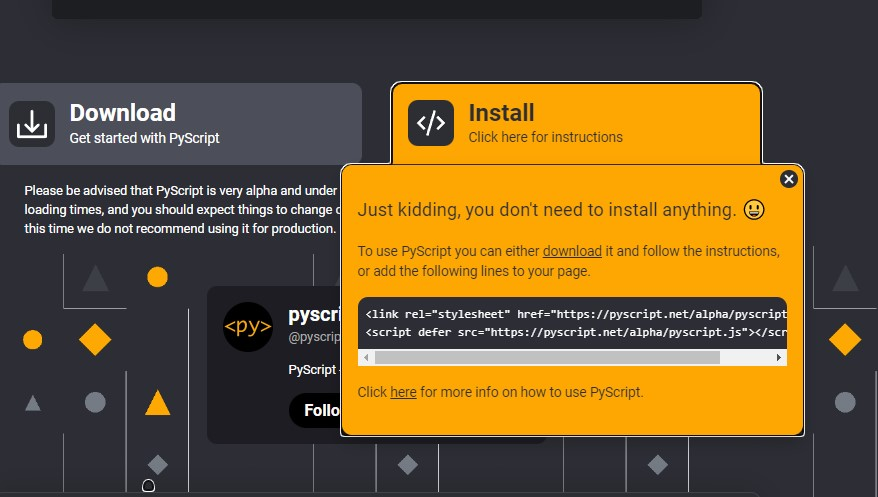pyscript site is just joking 😂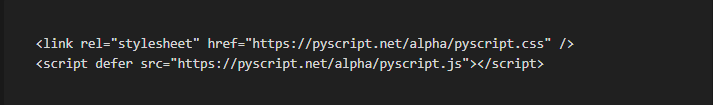must include files in head tag

Here I have a basic HTML code and in the head section we now paste this in so here we have the link to the style sheet which is a CSS file and then we include the script so this is py script.js and now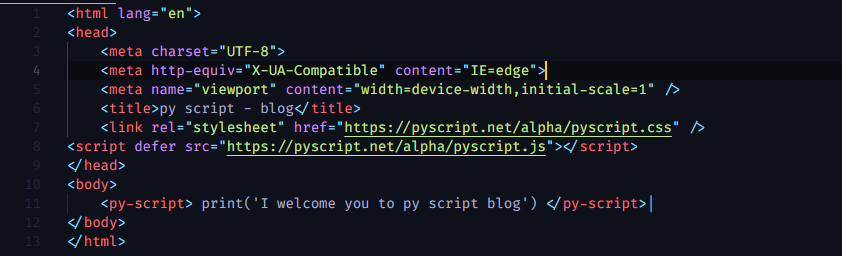code in pyscript

If we go back to the website we can grab this example script so now in the body here we can start the py script element and now here we can have any python code that we want so for example here we say print now you can and then we have the closing tags and this is all that we need so here I have visual studio code and I have the live server so now I can click on go live and this should start a website and now here we have the py script tests and now you can so it's printing what I print here so this is how to use it now let's have a look at a few more examples and you also find them on the official Github repository so this is open source and there you find a cool, getting started markdown file that you can check out,this is the 1st code run on browser

## Examples codes of py script

### Example no - 1

Now let's insert another py script element, and here we can define a function, for example, where we compute py, then we return py, then we call this function, and then again we print the result, so now let's save this and then go back to the website and if we refresh this then here we see py is approximately 3.142 so this works as well.

```print("Let's compute п:")
def wallis(n):
pi="2
for i in range(1,n):
pi * 4 * i ** 2 / (4* i *
return pi
pi = wallis (100000)
s = f"n is approximately {pi:.3f}"
print(s)```

### Example no - 2

so here are a few more examples so this is how we can set an HTML element afterward so we can give it an id, for example, today and then in a py script, we say py script. write and then we use this id and here we write the date of today so then here we again write the variable of py which is defined here and for this, we use diff with the id py and we also give it a class for styling so for this I included another script which is using bootstrap so now this is a class from bootstrap.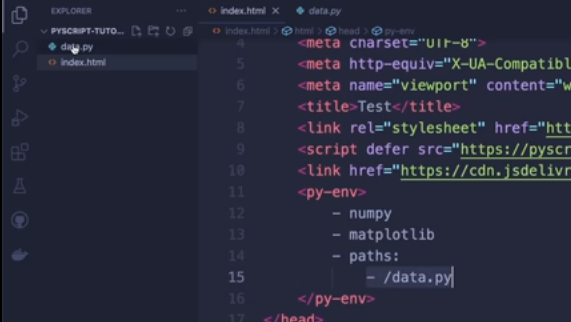main html file

let's also see how we can use matplotlib and how we can use a local file in order to use this at the top we define the py-env and here we define all the libraries we need so the scientific libraries like NumPy and Matlab clip are already included so we just have to list this here then we can list the paths and here we can list all the files that we want to include so here I created a local file data.py that is using NumPy and is creating two random arrays so now um let's again create another Num py script .

Here we have a new div with the id plot and here we say the output is a plot so this is where the plot will appear then we can import the libraries like in normal code we also import our function from the local data file then we create our data and then we say py. subplots and do what we want with matplotlib and then here we simply list the figure and now if we save this and go back to the file then this might take a few moments so it's still not the fastest but now here it works so here we have again this py with different styling and here we have our matplotlib.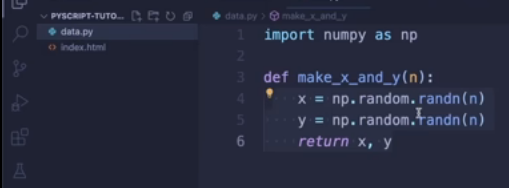data.py file

so yeah super cool now I want to show you one more cool feature and this is how easily we can use a rebel on our website so for this we just include this py rebel element and give it a id and then we can say auto generate equals true and if we refresh the website then here we have a wrapper and here we can run any python code that we want for example again print hello world and then I pressto shift enter and then it gets evaluated and here we see the output for example I can also import a module and then print .

here is the all output of the preview code

it is approximately 3.142matplotlib output using pyscript

### Example no - 3 Repl examples

let's say random number is 12 and then again shift enter and then here we have an output so this is super cool how easy we have a dynamic ripple on the website so how does this work for this I recommend visiting the anaconda engineering blog so in this post.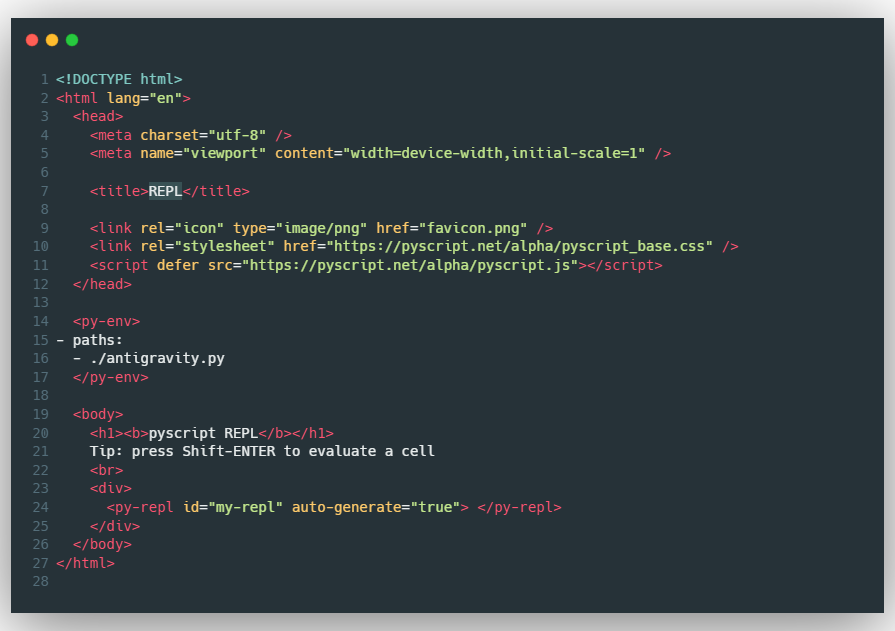code

So here is the another version of the Repl v2 on the py script the almost process is the same don’t worry I will provide the all the resources that I have been used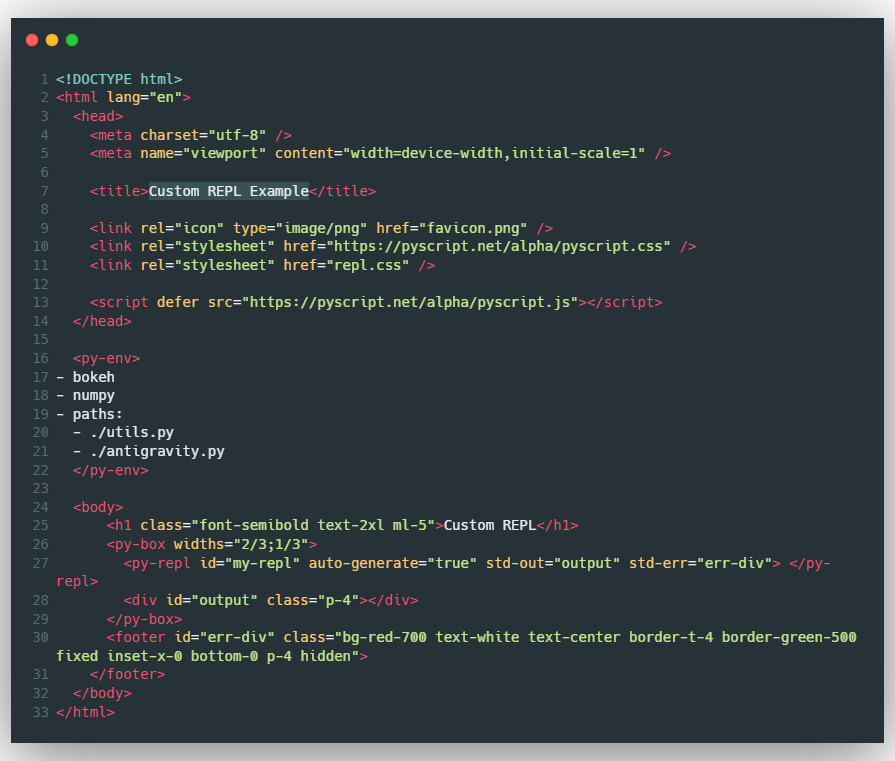Code

### All Examples

Simple Clock using PyScript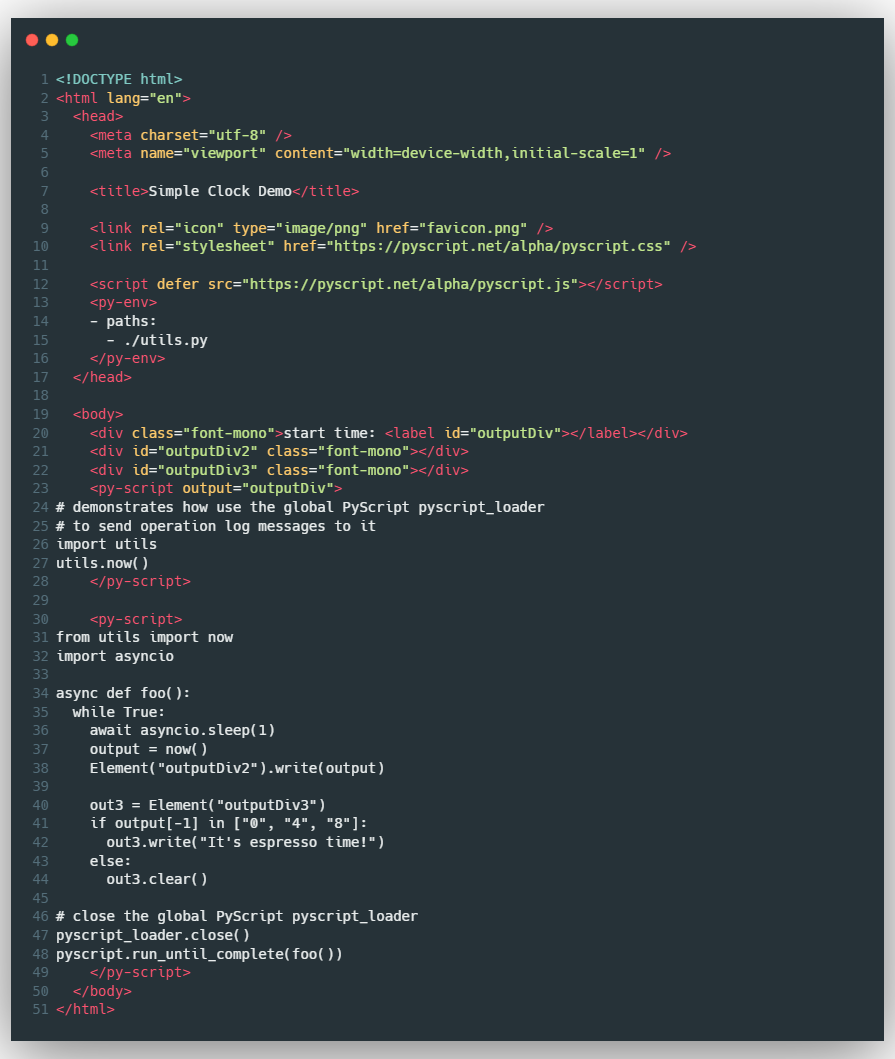code of the simple clock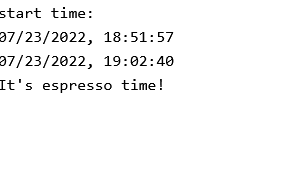output of the simple clock

Todo list using pyscript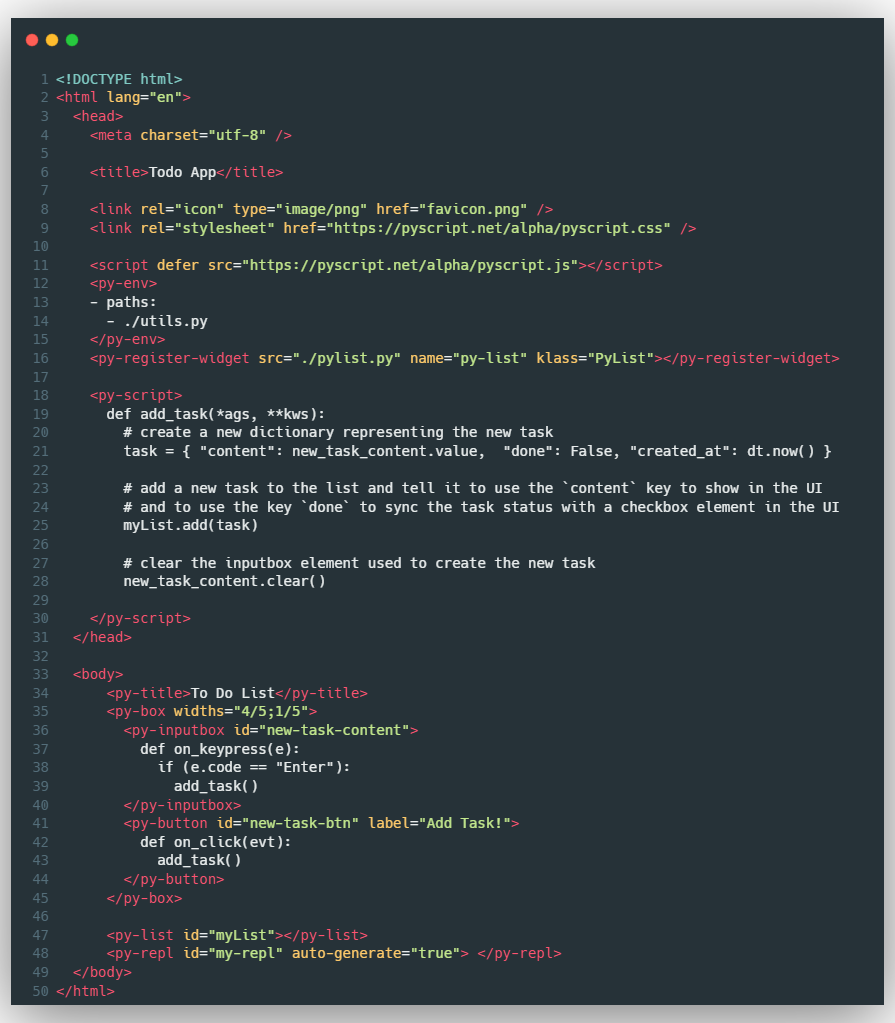code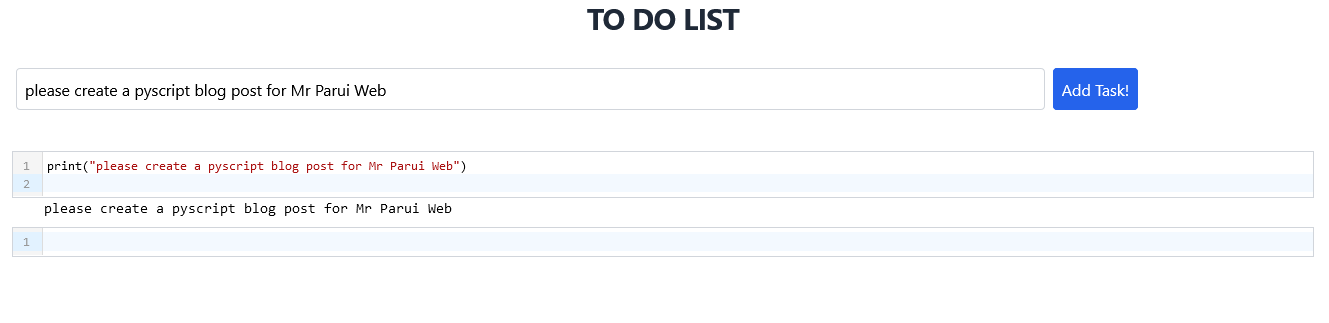output of todo list using PyScript

### play game with py script

Now, finally, let's play Mario. So yeah, Mario also is available in the py script repository under the example, so here you can check this out, and here you see that it combines the py script python code with javascript files. This is super cool.

I'd love to hear your thoughts on py script in the comments down below

## Conclusion

So If you are reading till here then you are a great reader like me and loves to read all the information that I have grind in this blog for you, also you invested the time to read the whole blog that’s amazing you know.

One last request if you liked my blog then you can share it with anybody, anywhere and anyone it would be appreciable also if you wish you can follow my social media handles and never miss any updates. Check it out

All resource that I have been used

Source:
www.mrparuiweb.dev

Hey ! 👋 Myself Pratap. I'm Graduate student, I talk & write about Frontend web development and some times Graphic Designs or UI/UX Designs. Subtly charming introvert, but I liked to share …

Created By

### Mr Parui Web.dev

All Posts are Protected By DMCA.
Reproduction in Any Form is Strictly Prohibited!